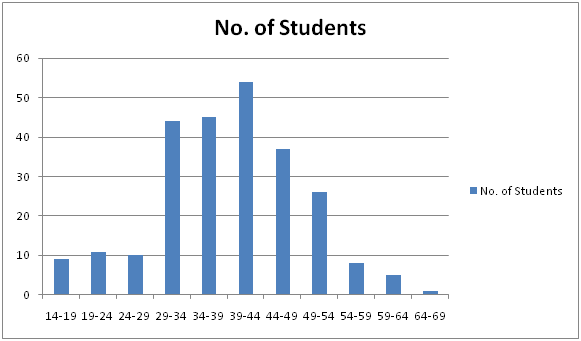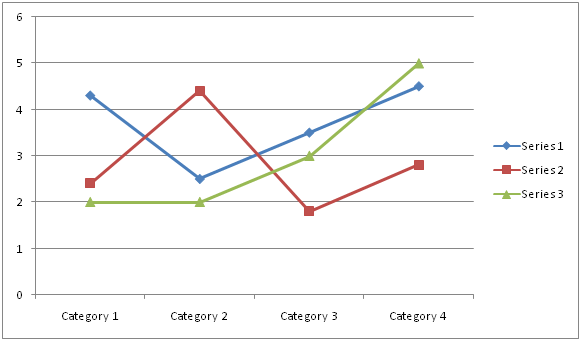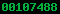﻿
Deprecated: mysql_connect(): The mysql extension is deprecated and will be removed in the future: use mysqli or PDO instead in /home/purvgwtw/public_html/tutorials/common.php on line 7
Descriptive Measures, Frequency DistributionHOME ABOUT US CONTACT US SERVICES
 Sponsered Links Categories Sponsered Links Amazon.com Widgets

# Descriptive Measures, Frequency Distribution

DESCRIPTIVE MEASURES

Quantitative data is a mass exhibit certain general characteristics or the way they are different from each are as follows:-

i.    Measures of Central Tendency or Averages
ii.   Measures of Variation or dispersion
iii.  Measures of skewness
iv.  Measures of Kurtosis

Frequency Distribution
When observation, discrete or continuous, are available on a single characteristics of a large number of individuals, often it become necessary to minimize or ease the data without losing it. Let us consider marks of 100 students in Statistics a follows:-

33    56    45    67    45
45    98    76    94    73
34    76    87    42    56
71    91    93    45    34
61    56    87    93    55
39    11    78    51    67
94    67    53    87    82
52    99    13    67    82
45    65    67    87    99
52    51    61    98    99
54    76    72    34    11
51    91    89    99    78
34    33    12    76    49
88    53    67    56    73
88    98    34    23    42
65    76    12    11    99
12    91    78    55    56
45    65    71    89    34
45    76    87    34    23
12    11    56    98    78

Understanding the above data is difficult, time consuming and confusing. A better way to present this data is in the form of Tally marks as shown below:-

Marks    Number of Students    Total Frequency
33                         II                                2
45                         IIII II                            7
34                         IIII II                            7
71                         II                                2
39                         I                                 1
94                         II                                2
52                         II                                2
54                         I                                 1
51                         III                               3
88                         II                                2
65                         III                               3
12                         IIII                              4
56                         IIII I                            6
98                         IIII                              4
76                         IIII I                            6
91                         III                               3
11                         IIII                              4
67                         IIII I                            6
99                         IIII                              5
53                         II                                2
93                         II                                2
87                         IIII                              5
78                         IIII                              4
13                         I                                 1
61                         II                                2
72                         I                                 1
89                         II                                2
42                         II                                2
23                         II                                2
55                         II                                2
82                         II                                2
49                         I                                 1
73                         II                                2

A Bar (1) is called a tally mark. Representation of the data in form of above shown manner is called frequency distribution. Here, Marks are called the variable (x) and the ‘number of students’ against the marks is known as the frequency of the variable.

Graphical Presentation of a Frequency Distribution

•     Histogram
•     Frequency Polygon

Histogram - Graphical representation of data in the form of rectangular continuous bars is called Histogram. It is used to represent data in such a way which is easy to understand and analyses.

Marks    No. of Students

14-19               9
19-24              11
24-29              10
29-34              44
34-39              45
39-44              54
44-49              37Note:- The upper and lower class limits of the new exclusive type are known as class boundaries.

Frequency Polygon - Graphical presentation of data, in case of ungrouped distribution, the frequency polygon is obtained by plotting points with abscissa as the variate value and the ordinates as the corresponding frequencies and joining the plotted points by the mean of straight line. For example one can see the below :-

In case of Ungrouped distribution

Category    Series 1    Series 2    Series 3
Category 1    4.3             2.4             2
Category 2    2.5             4.4             2
Category 3    3.5             1.8             3
Category 4    4.5             2.8             5In case of Grouped distribution - For equal class intervals the frequency polygon can be obtained by joining the middle points of the upper sides of the adjacent rectangles of the histogram by the mean of straight line.

Average (OR MEASURE OF CENTRAL TENDENCY) - Five measures of Central tendency that are in common use:-

1.     Arithmetic Mean or simple mean
2.    Median
3.    Mode
4.    Geometric Mean
5.    Harmonic Mean

Arithmetic Mean or simple mean - Arithmetic mean is set of observations is their sum divided by the number of observation.

Mean = 1 / n(x1+x2+x3+⋯+xn)

For example:- (a) Find the arithmetic mean of the following frequency distribution:
X:    1    2    3      4      5      6     7
Y:    5    9    12    17    14    10    6

(b) Calculate the arithmetic mean of the marks from the following table:-
Marks                0-10    10-20    20-30    30-40    40-50    50-60
No. of students    12       18         27         20         17         6

Solution:- (a) COMPUTATION OF MEAN
X        F         Fx
1        5         5
2        9         18
3        12       36
4        17       68
5        14       70
6        10       60
7        6        42
Total   73      299

Mean =  1 / N ∑fx = 299 / 73 = 4.09

(b) COMPUTATION OF MEAN

Marks    No. of students    Mid-point       Fx
0-10               12                   5              60
10-20             18                  15             270
20-30             27                  25             675
30-40             20                  35             700
40-50             17                  45             765
50-60              6                   55             330
Total             100                                  2800

Arithmetic mean =  1/N   ∑fx =  1/100 X2800 = 28

The arithmetic is reduced to a great extent by taking the deviation of the given values from any arbitrary point ‘A’ as explained below:

Let di = xi - A.    Then  fi di = fi (xi - A) = fi xi - Afi

Summing both sides over I from 1 to n, we get

fidi =∑fi xi  - A∑fi = ∑ fi xi – A.N

⇒1 / N∑fi di = 1 / N∑ fi xi  – A = Mean - A

Mean = A + 1 / N∑fi di

Properties of arithmetic Mean

•     Algebraic sum of the deviation of a set of values from their arithmetic mean is zero.
•     The sum of the square of the deviation of a set of values is minimum when taken about mean.
•     If xi ( i= 1,2,3,….k) are the mean of k component series of size n, ( i= 1,2,3,….k ) respectively, then mean x of the composite series obtained on combining the component series is given by formula:

x = (n1 x1  +n2 x2  + ……+nk xk) / (n1+ n2+⋯+nk)

= (∑ki=1ni  xi) / (∑ki=1 ni)

Example: The average salary of male employees in a firm was Rs 5200 and that of females was Rs 4200. The mean salary of all employees was Rs 5000. Find the percentage of male and female employees.

Solution: Let n1 and n2 denote respectively the number of male and female employees in the firm, and  x1 and  x2 denote respectively their average salary (in rupees). Let x denotes the average salary of all the workers in the firm.
We are given that: x1 = 5200,     x2 = 4200        and    x = 5000
Also we know
X = ( n1 x1  + n2 x2  ) / ( n1 + n2 )
= 5000 (n1+n2)
= 5200 n1 + 4200 n2
⇒ (5200 - 5000) n1  = (5000 - 4200) n2
⇒ 20n1 = 80n2
⇒ n1 / n2   = 4 / 1

Therefore, The percentage of male employees in the firm = 4/(4+1)  *100 = 80
And the percentage of female employees in the firm = 1/(4+1) *100 = 20

Example - The following is the age distribution of 1,000 person working in a large industrial house:
Age-group    No. of person
20-25                     30
25-30                     160
30-35                     210
35-40                     180
40-45                     145
45-50                     105
50-55                     70
55-60                     60
60-65                     40

Due to continuous heavy losses the management decides to bring down the strength to 30% of the present number according to the following scheme:-

•     To retrench the first 15% from the lower group.
•    To absorb the next 45% in other branches.
•    To make 10% from the highest age group retire permanently, if necessary.

Calculate the age limits of the persons retained and those to be transferred to other departments. Also find the average age of those retained.

Solution: Total number of person in the industrial house is N = 1000.
According to the conditions of the problem: The number of persons to be retrenched from the lower group
= 15 % of N =15 / 100 * 1000 = 150.
30 of these will be from first group i.e 20 - 25 and remaining 150 - 30 =120, from the next age group 25 - 30.
The number of persons  to be absorbed in other branches:
= 45% of N = 45 / 100 * 1000 = 450.

These will belong to different age group as detailed below:-
Age-group    Number of persons
25-30                  160-120* = 40
30-35                   210
35-40                   180
40-45                   450 - (40+210+180) = 20
[*: Because 120 persons from this group have been retrenched in i.]

The number of persons to retire(from the highest age groups)
= 10% of N =10 / 100 * 1000 =100

These 100 persons are from the highest age-groups as shown:
Age-group    Number of persons
55-60                          60
60-65                          40

Hence incorporating the steps in (i), (ii) and (iii),the frequency distribution of the number of persons retained in the industrial house is as shown in the adjoining table:
Age-group    Number of persons
40-45                     145-20 = 125
45-50                     105
50-55                      70

CALCULATION FOR AVERAGE AGE OF THOSE RETAINED

Age-group    Mid-value     f               d= (x-47.5)/5        Fd

40-45                42.5          125                   -1                -125
45-50                47.5          105                    0                0
50-55                52.5          70                      1                70
Total                                 300                                      55

The average age of those retained is given by:-
X  =  Mean = A+ 1 / N∑fi d= 47.5 + (5*(-55))/300 =46.5833 => 47
Hence, the average age of those retained in the industrial house is 47 years.

MERITS AND DEMERITS OF ARITHEMATIC MEAN

Merits

1. It is rigidly defined.
2. It is easy to understand as well as to calculate.
3. It is based on all observation
4. It is amenable to algebraic treatment
5. Among all the averages, arithmetic mean is affected least by fluctuation of sampling. It is also known as stable average.

Demerits

1. It cannot be determined by inspection nor can be graphically represented.
2. Cannot not be used when dealing with qualitative data for example-honesty, intelligence, etc.
3. It cannot be calculated even if a single value is missing or illegible un less we drop it out and compute the arithmetic mean with remaining values.
4. It is affected very much by extreme values. In case of extreme values arithmetic mean gives a distorted image of the distribution and no longer remains representative of the distribution.
5. It may lead to wrong conclusions if the details of the data from which it is computed are not given.

Weighted Mean
We all know that each and everything have their own importance for example commodities. Not all commodities have equal importance in our life like wheat, rice and pulses are more important as compared to cigarette, tea, confectionary, etc.
In real time scenarios while calculating mean as per the importance weights are given to commodities.

For Example:-
Find the simple and weighted arithmetic mean of the first n natural numbers, the weights being the corresponding numbers.
Solution:- The first n natural numbers are:-        1, 2, 3, ……n.
X        w        wX
1        1          12
2        2          22
3        3          32
-         -           -
-         -           -
n        n          n2

We know that

1 + 2 + 3 + ……+n =(n(n+1))/2

12 + 22 + 32 + …..+ n2 = (n(n+1)(2n+1))/6

Simple A.M = (∑X)/n =  (1+2+3+⋯+n)/n = (n+1)/2

Weighted A.M = (∑wX)/(∑w) = (12  + 22  + 32  + …..+ n2)/(1 + 2 + 3 + ……+n ) = (n(n+1)(2n+1))/6 * 2/(n(n+1))
= (2n+1)/3

 Sponsered Links Latest Updates
 All Content of this site is for learning only. We do not warrant the correctness of its content. The risk from using it lies entirely with the user. While using this site, you agree to have read and accepted our terms of use and privacy policy. Copyright © 2014 PURVACOMPUTERS.COM All Right Reserved